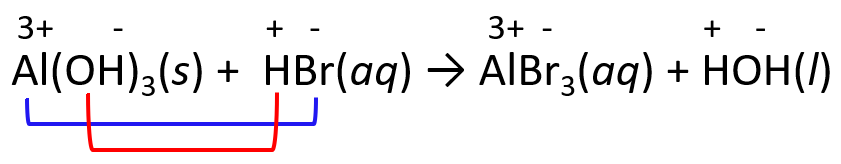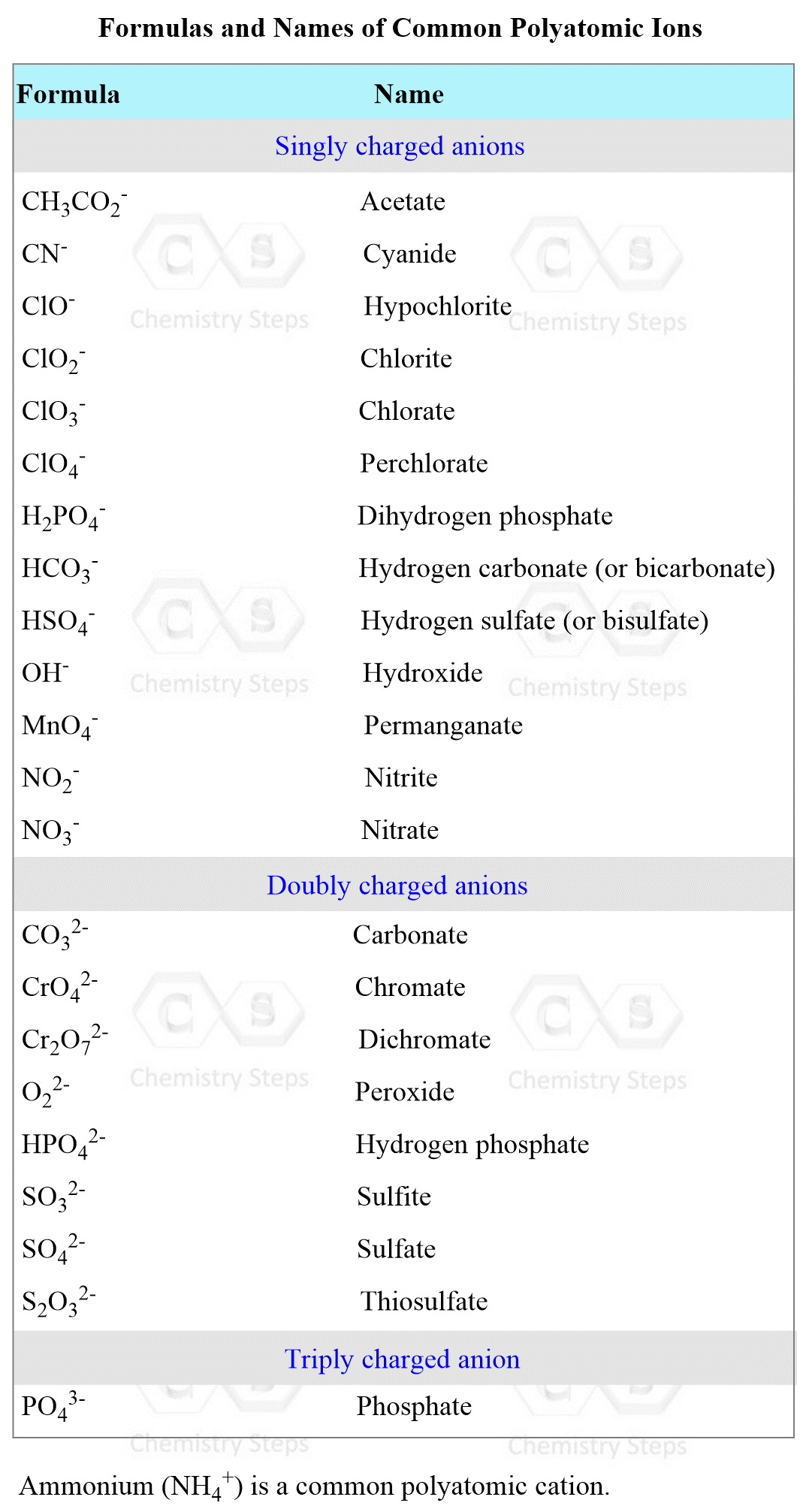## General Chemistry

In the previous post, we talked about the definition of acids and bases according to the Arrhenius, Brønsted–Lowry, and Lewis acid-base theories. Refresh these concepts if you need to, but most often, you are going to identify acids as compounds with an H, and bases as the ones containing an OH group.

Now, acid-base reactions are an important class of reactions in chemistry known also as neutralization reactions.

For example, the reaction between hydrochloric acid (HCl) and sodium hydroxide (NaOH) produces sodium chloride (NaCl) and water (H2O):

HCl(aq) + NaOH(aq) →  NaCl(aq) + H2O(l)

Sodium chloride is the table salt, and this is a general pattern for acid-base reactions to produce a salt and water.

acid + base → salt + water

For example,

3H2SO4(aq) + 2Al(OH)3(aq)  → Al2(SO4)3(aq)  + 3H2O(l)

2HNO3 + Ba(OH)2 → Ba(NO3)2 + 2H2O(l)

Like the precipitation reactions, acid-base reactions are another type of metathesis of double-displacement reactions.

Remember, these reactions occur by an exchange of ions, so for an acid-base reaction, the proton from the acid is combined with the anion of the base and the cation of the base is combined with the anion of the acid.

For example, in the reaction between HBr and Al(OH)3, we predict the products by exchanging the ions.Notice that water is formed by combining the H+ and OH- ions which gives HOH and this is another representation of water.

To be able to predict the products of a double displacement reaction, you need to know the formulas and the charges of the ions in the starting materials.

The most common polyatomic ions are shown in the table below:# Ionic and Net Ionic Equations for an Acid-Base Reaction

Remember, when discussing the molecular, ionic, and net ionic equations, we said that the ionic equation is when we show the electrolyte dissociated into ions, and in the net ionic equation, we omit the spectator ions.

For example,

Ionic equation:

6K+(aq) + 2PO43-(aq) + 3Ba2+(aq) + 6Cl(aq→ Ba3(PO4)2(s) + 6K+(aq) + 6Cl(aq)
Spectator ions

Net ionic equation:

3Ba2+(aq) + 2PO43-(aq) → Ba3(PO4)2(s)

Now, for acid-base reactions, we rely on the same principle of dissociating the electrolytes and leaving the solids, liquids, and gases intact.

For example,

HCl(aq) + NaOH(aq) →  NaCl(aq) + H2O(l) – Molecular

H+(aq) + Cl(aq) + Na+(aq) + OH(aq) → Na+(aq) + Cl(aq) + H2O(l) – Ionic

Spectator ions

H+(aq) + OH(aq) → H2O(l) – Net ionic

Weak acids such as HCN, HF, and CH3CO2H (acetic acid) are not dissociated either.

For example,

HCN(aq) + NaOH(aq) →  NaCN(aq) + H2O(l) – Molecular

HCN(aq) + Na+(aq) + OH(aq) → Na+(aq) + CN(aq) + H2O(l) – Ionic

Spectator ions

HCN(aq) + OH(aq) → CN(aq) + H2O(l)  – Net ionic

# Acid-Base Reactions Leading to Gas Formation

Some salts containing ions such as carbonates (CO32- ion), bicarbonates (HCO3 ion), sulfites (SO32-), and sulfides (S2-) produce gaseous products when reacted with acids.

For example, hydrochloric acid readily reacts with baking soda producing NaCl, carbon dioxide (CO2), and water.

HCl(aq) + NaHCO3(aq) → NaCl(aq) + H2O(l) + CO2(g)

What happens here is that the H+ and HCO3 ions combine to form carbonic acid (H2CO3) which is very unstable and immediately decomposes to CO2 and H2O:

H2CO3 → CO2 + H2O

The overall reaction between HCl and NaHCO3 lists CO2 and H2O as final products without showing the carbonic acid.

The ionic and net ionic equations for this reaction would be:

H+(aq) + Cl(aq) + Na+(aq) + HCO3(aq) → Na+(aq) + Cl(aq) + H2O(l) + CO2(g)- Ionic

Spectator ions

H+(aq) + HCO3(aq) → H2O(l) + CO2(g) – Net ionic

In a similar way, sulfites and sulfides produce H2S and SO2 gases respectively:

Na2SO3(aq) + 2HCl(aq) → 2NaCl(aq) + H2O(l) + SO2(g)

K2S(aq) + 2HCl(aq) → 2KCl(aq) + H2S(g)

# Lewis Acid-Base Reactions

Another type of acid-base reaction is the one between Lewis acids and bases. Remember these are classified based on their electron donor (base) and acceptor (acid) properties.

For example, methylamine (CH3NH2) is a derivative of ammonia, and it acts as a base when reacted with acid by donating its lone pair of electrons on the nitrogen:The HCl is the acid because; 1) it accepts the electrons from the nitrogen, 2) it is a proton donor, so it is still a Brønsted acid.

Now, if we replace the HCl with borane (BH3), we cannot classify it as an acid, by the Arrhenius or Brønsted theory because there is no proton involved in the reaction:

CH3NH2      +      BH3              (CH3NH2)+-(BH3)
base                acid                           salt

What is happening is that the nitrogen donates in lone pair of electrons to boron which can accept it because of an empty orbital.

The acid and base in this reaction can only be classified based on the electron transfer between them and, therefore, we can only identify the acid and the base by the Lewis theory.

Check Also

#### Practice

1.

Acid rain containing HNO3 reacts with CaCO3 and accelerates decaying of statues. Write the net ionic equation for this reaction.